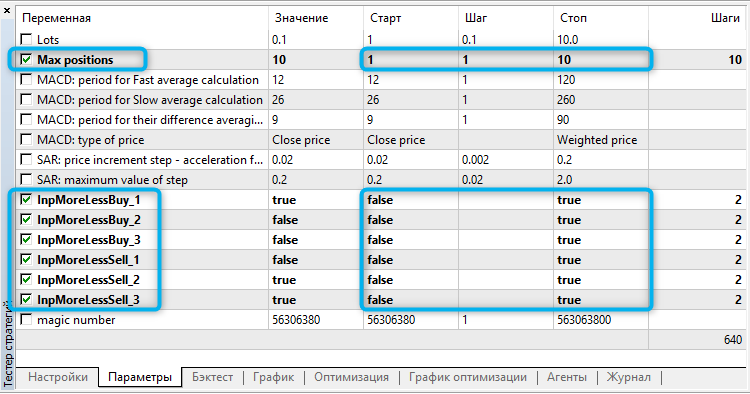# MACD and SAR – expert for MetaTrader 5

• A+

Idea by: Gatis.

MQL5 code by: Vladimir Karputov.

The EA analyzes four parameters:

1. Value of the main line of MACD on bar #1 (macd_main_1)
2. Value of the signal line of MACD on bar #1 (macd_signal_1)
3. Value of indicator SAR on bar #1 (sar_1) relative to price Bid (m_symbol.Bid())

These parameters are combined in the basic formula of signals for Buy and Sell:

```bool open_buy        = (macd_main_1>macd_signal_1 && macd_signal_1<0 && sar_1<m_symbol.Bid());
bool open_sell       = (macd_main_1<macd_signal_1 && macd_signal_1>0 && sar_1>m_symbol.Bid());
```

However, you can optimize the values of signs < and > in any part of the formula. To do so, for each of signs < and >, their own variables (InpMoreLessBuy_1, InpMoreLessBuy_2, InpMoreLessBuy_3, InpMoreLessSell_1, InpMoreLessSell_2, and InpMoreLessSell_3) are introduced. With these variables, the basic formula is modified as follows:

```bool open_buy=    (InpMoreLessBuy_1   ? macd_main_1>macd_signal_1      : macd_main_1<macd_signal_1) &&
(!InpMoreLessBuy_2  ? macd_signal_1 < 0              : macd_signal_1 > 0 ) &&
(!InpMoreLessBuy_3  ? sar_1         < m_symbol.Bid() : sar_1         > m_symbol.Bid() );
bool open_sell=(!InpMoreLessSell_1 ? macd_main_1<macd_signal_1      : macd_main_1>macd_signal_1) &&
(InpMoreLessSell_2  ? macd_signal_1 > 0              : macd_signal_1 < 0 ) &&
(InpMoreLessSell_3  ? sar_1         > m_symbol.Bid() : sar_1         < m_symbol.Bid() );
```

The EA itself only operates when a new bar appears. When a signal is received, positions opposite to the signal received will be closed.

When optimizing the formula, it would also be reasonable to optimize the number of positions: